# 4.4: Right Triangle Trigonometry

•• Contributed by CK12
• CK12

Trigonometry is the study of triangles. If you know the angles of a triangle and one side length, you can use the properties of similar triangles and proportions to completely solve for the missing sides.

Imagine trying to measure the height of a flag pole. It would be very difficult to measure vertically because it could be several stories tall. Instead walk 10 feet away and notice that the flag pole makes a 65 degree angle with your feet. Using this information, what is the height of the flag pole?

### Trigonometric Functions

The six trigonometric functions are sine, cosine, tangent, cotangent, secant and cosecant. Opp stands for the side opposite of the angle $$\theta,$$ hyp stands for hypotenuse and adj stands for side adjacent to the angle $$\theta$$.

\begin{aligned} \sin \theta &=\frac{o p p}{h y p} \\ \cos \theta &=\frac{a d j}{h y p} \\ \tan \theta &=\frac{o p p}{a d j} \\ \cot \theta &=\frac{a d j}{o p p} \\ \sec \theta &=\frac{h y p}{a d j} \\ \csc \theta &=\frac{h y p}{o p p} \end{aligned}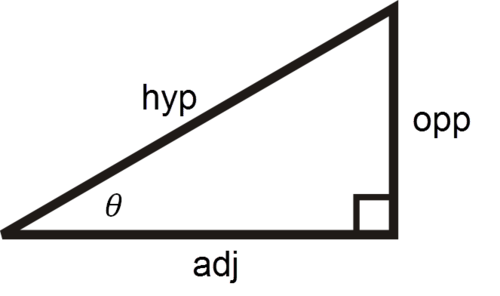The reason why these trigonometric functions exist is because two triangles with the same interior angles will have side lengths that are always proportional. Trigonometric functions are used by identifying two known pieces of information on a triangle and one unknown, setting up and solving for the unknown. Calculators are important because the operations of sin, cos and tan are already programmed in. The other three (cot, sec and csc) are not usually in calculators because there is a reciprocal relationship between them and tan, cos and sec.

$$\sin \theta=\frac{o p p}{h y p}=\frac{1}{\csc \theta}$$
$$\cos \theta=\frac{a d j}{h y p}=\frac{1}{\sec \theta}$$
$$\tan \theta=\frac{o p p}{a d j}=\frac{1}{\cot \theta}$$

Keep in mind that your calculator can be in degree mode or radian mode. Be sure you can toggle back and forth so that you are always in the appropriate units for each problem.

Note that the images throughout this concept are not drawn to scale. If you were given the following triangle and asked to solve for side $$b$$, you would use sine to find $$b$$.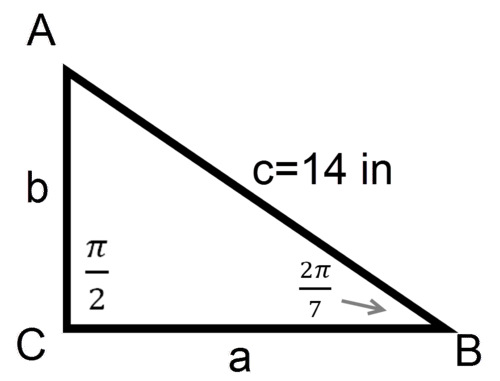\begin{aligned} \sin \left(\frac{2 \pi}{7}\right) &=\frac{b}{14} \\ b &=14 \cdot \sin \left(\frac{2 \pi}{7}\right) \approx 10.9 \mathrm{in} \end{aligned}

### Examples

Example 1

Earlier, you were asked about the height of a flagpole that you are 10 feet away from. You notice that the flag pole makes a $$65^{\circ}$$ angle with your feet.

If you are 10 feet from the base of a flagpole and assume that the flagpole makes a $$90^{\circ}$$ angle with the ground, you can use the following triangle to model the situation.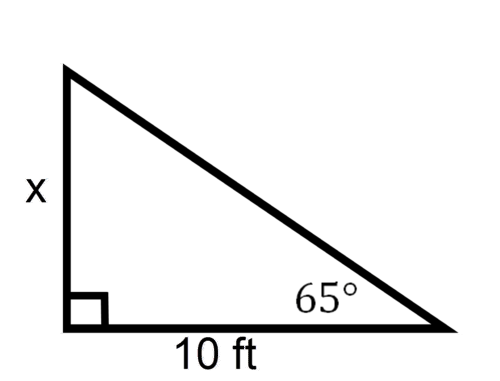\begin{aligned} \tan 65^{\circ} &=\frac{x}{10} \\ x &=10 \tan 65^{\circ} \approx 21.4 f t \end{aligned}

Example 2

Solve for angle $$A$$.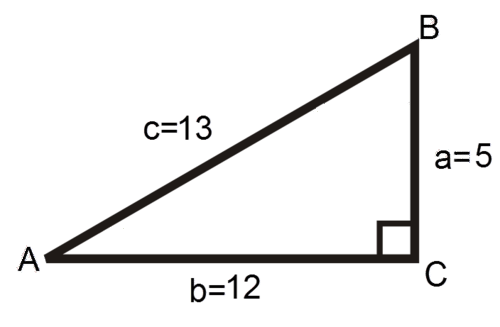This problem can be solved using sin, cos or tan because the opposite, adjacent and hypotenuselengths are all given.

The argument, or input, of a sin function is always an angle. The arcsin, or $$\sin ^{-1} \theta,$$ function on the calculator has an argument that is a ratio of the triangle sides.

\begin{aligned} \sin A &=\frac{5}{13} \\ \sin ^{-1}(\sin A) &=\sin ^{-1}\left(\frac{5}{13}\right) \\ A &=\sin ^{-1}\left(\frac{5}{13}\right) \approx 0.39 \text { radian } \approx 22.6^{\circ} \end{aligned}

Example 3

Given a right triangle with $$a=12$$ in $$, m \angle B=20^{\circ},$$ and $$m \angle C=90^{\circ}$$, find the length of the hypotenuse.

It is helpful to draw a diagram to represent the data given in a question.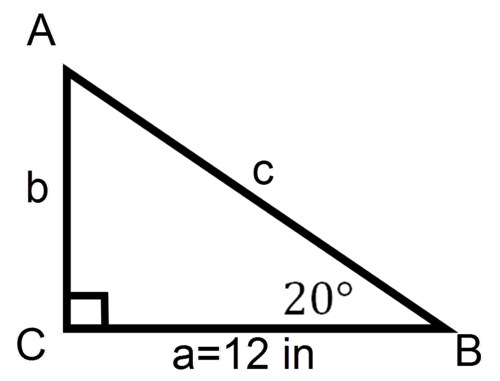\begin{aligned} \cos 20^{\circ} &=\frac{12}{c} \\ c &=\frac{12}{\cos 20^{\circ}} \approx 12.77 \mathrm{in} \end{aligned}

Example 4

Given $$\triangle A B C$$ where $$B$$ is a right angle, $$m \angle C=18^{\circ},$$ and $$c=12 .$$ What is $$a$$ ?

Drawing out this triangle, it looks like: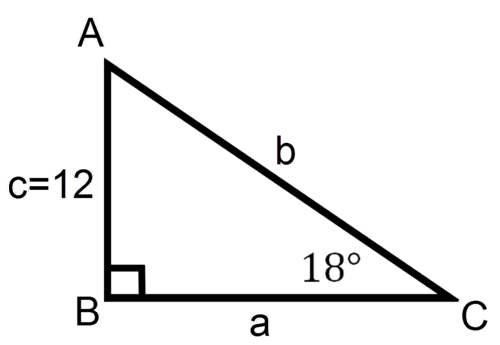\begin{aligned} \tan 18^{\circ} &=\frac{12}{a} \\ a &=\frac{12}{\tan 18^{\circ}} \approx 36.9 \end{aligned}

Example 5

Given $$\triangle M N O$$ where $$O$$ is a right angle, $$m=12$$, and $$n=14$$. What is the measure of angle $$M$$ ?
Drawing out the triangle, it looks like: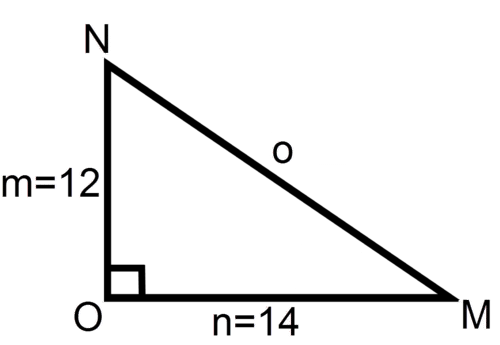\begin{aligned} \tan M &=\frac{12}{14} \\ M &=\tan ^{-1}\left(\frac{12}{14}\right) \approx 0.7 \text { radian } \approx 40.6^{\circ} \end{aligned}

Review

For $$1-15$$, information about the sides and/or angles of right triangle $$A B C$$ is given. Completely solve the triangle (find all missing sides and angles) to 1 decimal place.

 Problem Number $$A$$ $$B$$ $$C$$ $$a$$ $$b$$ $$c$$ 1. $$90^{\circ}$$ 4 7 2. $$90^{\circ}$$ $$37^{\circ}$$ 18 3. $$90^{\circ}$$ $$15^{\circ}$$ 32 4. $$90^{\circ}$$ 6 11 5. $$90^{\circ}$$ $$12^{\circ}$$ 19 6. $$90^{\circ}$$ 17 10 7. $$90^{\circ}$$ $$10^{\circ}$$ 2 8. $$4^{\circ}$$ $$90^{\circ}$$ 0.3 9. $$\frac{\pi}{2}$$ radian 1 radian 15 10. $$\frac{\pi}{2}$$ radian 12 15 11. $$\frac{\pi}{2}$$ radian 9 14 12. $$\frac{\pi}{4}$$ radian $$\frac{\pi}{4}$$ radian 5 13. $$\frac{\pi}{2}$$ radian 26 13 14. $$\frac{\pi}{2}$$ radian 19 16 15. $$\frac{\pi}{2}$$ radian 10 $$10 \sqrt{2}$$